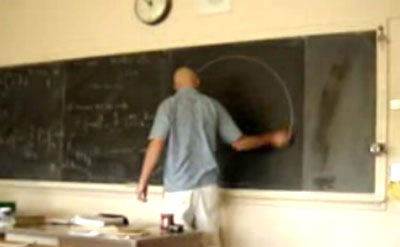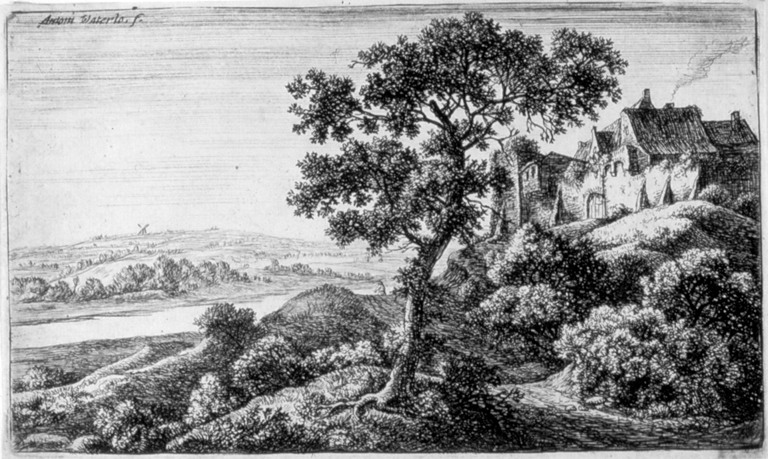Drawing Machine

(diff) ← Older revision | Latest revision (diff) | Newer revision → (diff)

Striving for the Pope's Commission

• Giotto's perfect circle.• Hand and Eye
• Legends: the high school teacher• linear apparatus. substructure.• Stroke recording apparatus. Two pulleys. Weights. Rotary encoders.

Simultaneous Recording and Reproduction

• Simultaneous Location And Perversion (SLAP)

Drawing Machine As Printmaking

• Harvey Moon
••• Antonie Waterloo
••DynaCNC Drawing Machine

do a john henry with the dynaCNCPen HolderHanging Plotter

do a john henry with the hanging plotter

Drawing Machine in SketchbookArticulated Arm

do a john henry with the disembodied hand.

disembodied hand

Imprecision as painterly aesthetic.Aikon by Frédéric Fol Leymarie and Patrick Tresset.

Thoughts

no such thing as perfect repetition. no matter what, there is noise in the system.

Pantomime / Mirror

GRBL1 fast

```\$0=7.023 (x, step/mm)
\$1=7.023 (y, step/mm)
\$2=7.023 (z, step/mm)
\$3=10 (step pulse, usec)
\$4=25400.000 (default feed, mm/min)
\$5=45720.000 (default seek, mm/min)
\$6=56 (step port invert mask, int:00111000)
\$7=25 (step idle delay, msec)
\$8=800.000 (acceleration, mm/sec^2)
\$9=0.050 (junction deviation, mm)
\$10=0.100 (arc, mm/segment)
\$11=25 (n-arc correction, int)
\$12=3 (n-decimals, int)
\$13=1 (report inches, bool)
\$14=1 (auto start, bool)
\$15=0 (invert step enable, bool)
\$16=0 (hard limits, bool)
\$17=1 (homing cycle, bool)
\$18=0 (homing dir invert mask, int:00000000)
\$19=400.000 (homing feed, mm/min)
\$20=10000.000 (homing seek, mm/min)
\$21=100 (homing debounce, msec)
\$22=1.000 (homing pull-off, mm)
```

GRBL1 moderate

```fill in here
```

grbl v 0.9a

```\$0=7.023 (x, step/mm)
\$1=7.023 (y, step/mm)
\$2=7.023 (z, step/mm)
\$3=500.000 (x v_max, mm/min)
\$4=500.000 (y v_max, mm/min)
\$5=500.000 (z v_max, mm/min)
\$6=10.000 (x accel, mm/sec^2)
\$7=10.000 (y accel, mm/sec^2)
\$8=10.000 (z accel, mm/sec^2)
\$9=10 (step pulse, usec)
\$10=20000.000 (default feed, mm/min)
\$11=56 (step port invert mask, int:00111000)
\$12=25 (step idle delay, msec)
\$13=0.050 (junction deviation, mm)
\$14=0.005 (arc tolerance, mm)
\$15=3 (n-decimals, int)
\$16=0 (report inches, bool)
\$17=1 (auto start, bool)
\$18=0 (invert step enable, bool)
\$19=0 (hard limits, bool)
\$20=0 (homing cycle, bool)
\$21=0 (homing dir invert mask, int:00000000)
\$22=25.000 (homing feed, mm/min)
\$23=250.000 (homing seek, mm/min)
\$24=100 (homing debounce, msec)
\$25=1.000 (homing pull-off, mm)
```